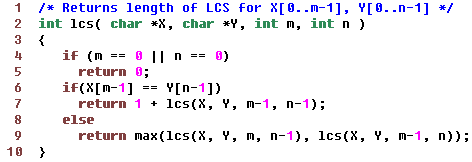Data Structuresand Algorithms Good OldJava AdvancedInterview Topics Cloud andDatabases Web Designand Development Must-knowTools Good ToKnow

## Longest common subsequence in 2 strings

The subsequence need not be contiguous.

Consider the order for brute force approach.

If a string has length n, then it will have 2n substrings.

So, if 2 strings are of length m and n, then comparison of all their substrings will be O(2m+n)

### Recursive formulationIn the above, we match characters from the end.

In each recursive step, we reduce the length of one of the strings when characters do not match. If characters match, then we reduce lengths of both the strings.

Overlapping subproblems: In the above case, several cases are calculated repeatedly which indicates a candidate for DP.

Example, if end of both the strings do not match, then 2 recursive calls in 1st step are:

1. n-1 character string and m character string.

2. n character string and m-1 character string.

In the recursions of each of the above, a call is generated for n-1 char string and m-1 string.

If we had stored the result of these computations, then it would have been much more efficient.

### Dynamic Programming Solution

We will need a matrix of size M+1 x N+1 for a lookup table.

If one of the strings has 0 length, then for all lengths of other string, max matching subsequence has 0 length.

If both the strings are of 1 length, then lookup will be either 0 or 1

If both the strings are of length 2, then entries possible are:

1. lookup = 1 or 0

2. lookup = 1 or 0

3. lookup = 1 or 0

4. lookup = 2, 1, or 0Note: The above needs a space complexity of O(mn) but our algorithm only needs values from a previous row. So we can optimize it by keeping just 2 rows at a time. When one lookup row is completely formed, we overwrite the previous row with the new row.

### Find subsequence also alongwith the length

This can be done by making a second pass through both the strings as follows:

```String findMaxSubsequence (String A, String B)
{
String S = "";
int i=0, j=0;
while (i < A.length && j < B.length)
{
if (A[i]==B[j])
{
S += A[i];
i++; j++;
}
else if (L[i+1,j] >= L[i,j+1]) i++;
else j++;
}
}
```

### Maximum common substring

Substring is contiguous while subsequence is not.
A string of length n has 2n subsequences and n(n+1)/2 substrings.

One good part of substring match problem is that once a non-matching character is found in the
strings, we can completely forget about the strings matched till those characters.
 ```int maxCommonSubstring (char[] X, char[] Y, int m, int n) { if (m < 0 || n < 0) return 0; int maxA = 0; if (X[m] == Y[n]) { // If one char has matched, check how far the match goes while (m >= 0 && n >= 0 && X[m] == Y[n]) { maxA++; m--; n--; } } int maxB = maxCommonSubstring (X, Y, m-1, n); int maxC = maxCommonSubstring (X, Y, m, n-1); return max (maxA, maxB, maxC); } ``` This solution will compare each substring with each other substring. Total comparisons for strings of lengths m and n would be:     m(m+1)/2 * n(n+1)/2 Right hand side proves that for m=3, n=3, total recursive calls are: 3*4/2 * 3*4/2 = 36 It is easy to see that lots of subproblems are being calculated again and again. And the order can be improved by using dynamic programming here. Sample Execution for worst case ```abc, def ab, def a , def "", def a , de "", de a , d "", d a, "" ab, de a , de "", de a , d "", d a, "" ab, d a , d ab, "" abc, de abc, d abc, "" ab, d a , d "", d a, "" ab, "" ab, de a , de "", de a , d ab, d a , d "", d a, "" ab, "" ```

Like us on Facebook to remain in touch
with the latest in technology and tutorials!

Got a thought to share or found a
bug in the code?
We'd love to hear from you:

 Name: Email: (Your email is not shared with anybody) Comment: